Disclaimer: This is an example of a student written essay.

Any scientific information contained within this essay should not be treated as fact, this content is to be used for educational purposes only and may contain factual inaccuracies or be out of date.

# Analysis of Efficiency of Solar PV Cell

 ✅ Paper Type: Free Essay ✅ Subject: Sciences ✅ Wordcount: 1452 words ✅ Published: 10th Apr 2018

The efficiency estimation is the most frequently method used to compare the performance of solar cells. This method can be defined as the ratio of power output from the solar cell over the irradiance power output. In addition of estimating the performance of the solar cell itself, the efficiency depends on the spectrum, intensity of sunlight and the temperature of the solar cell (Fouladi et al., 2013). The efficiency of solar cells can be estimated using the following Equation 3.15:

η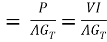Where I is the output current, V is the voltage, A is the aperture surface area of the PV module. P is the electrical power generated, GT is the solar radiation flux on module plane (W/m2) and η is the cell/module electrical efficiency. The panel efficiency is effected by the cell temperature and irradiance and this effect in efficiency can by estimated by connecting the existing environment conditions to a reference condition using the following Equation 3.16 (Fouladi et al., 2013):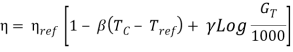ηref is the cell efficiency at the reference temperature, Tref (typically 25 ºC), 1000 W/m2 is the reference solar irradiance. β is the temperature coefficient and γ is the solar irradiance coefficient and both coefficients depend on the PV materials.

From Equation 3.16, it can be noticed that an increase in cell temperature (TC) would result in decrease in efficiency (Fouladi et al., 2013). The value of γ is often assumed to be zero and Equation 3.17 will be: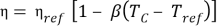ηref and β values are given by the manufacturers. However, the can be estimated using a flash test in which the cell’s electrical output is measured at two different temperatures for given solar radiation flux (Hart and Raghuraman, 1982; Skoplaki and Palyvos, 2009). The actual value of β it depends on Tref along with the dependence on PV materials and it can be given by Equation 3.18: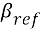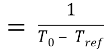T0 is the temperature at which the electrical efficiency of the PV module reach is zero (Garg and Agarwal, 1995; Skoplaki and Palyvos, 2009). For crystalline silicon solar cells the highest temperature in at which the PV module electricity drops to Zero is 270 ºC (Evans and Florschuetz, 1978; Skoplaki and Palyvos, 2009).

The output voltage decreases significantly with the increase of the cell temperature and that causes the current to increase slightly. Nominal operation cell temperature method (NOCT) is used to estimate the cell temperature. This method estimates the cell temperature by measure the cell temperature of an open-rack PV module under an open circuit and in a standard reference environment Fouladi et al., 2013).

The solar radiation is estimated typically to be 800W/m2 (GT, NOCT) and the PV panel is tilted 45º from the horizontal and exposed to 20 ºC air (Ta, NOCT) at 1 m/s. Equation 3.19 can be expressed as (Fouladi et al., 2013):

Tc =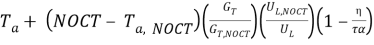Where UL is the thermal loss coefficient, Ta is the air temperature and GT is the irradiance on the plane of the panel. Coefficient τ is the solar transmittance of glazing and coefficient α is the solar absorptance of the PV layer. η is the electrical efficiency. According to (Duffie and Beckham, 1980; Fouladi et al., 2013), the term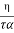is negligible so Equation 3.20 will become:

Tc =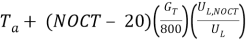UL which is the overall heat loss for the panel can be estimated using the following Equation 3.20:

UL =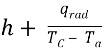Where h is the convective heat loss coefficient and qrad is the radiation heat loss from the panel.

There are other environmental factors that can influence the performance and the efficiency of the PV cells other than the temperature such as (Mekhilef et al., 2012):

1. Dust

Dust accumulation on the surface of PV panel reduces the amount of irradiance required for PV cell to reach maximum efficiency. The excessive accumulation of dust decreases the quality of the solar cells.

1. Humidity:

The effect of the humidity it is somehow comparable to the effect of the dust. The water vapor particles decreases the level of the sunlight irradiance needed for the PV panel to reach the maximum efficiency. When the PV surface becomes moist, the light is scattered either by reflection, diffraction or refraction when it comes in contact with the water droplets.

Beside the environmental factors that affect the efficiency of the PV panel, there are technical factors that are based on electricity generation system that also can influence the efficiency of the module such as (Darwish et al., 2013):

1. Effect of tilt angle:

The tilt angle is one of major factors that determine the performance of the PV panels. The variation of the angle that the system is set with can control the level of the dust disposition on the glass and the amount of irradiance transmission of the plate under various weather conditions.

1. Effect of PV Technology

Photovoltaic technology is classified into two groups which are silicon crystalline and thin film. Each group of PV modules consists of different types of solar cells. These different types of solar cell vary also in their efficiencies, resistance to high temperatures and also resistance to harsh environment locations. The investigations found that a-Si performs best in dusty environment.

1. Effect of Cleaning

There are four methods to remove the dust and reduce the effect of the dust on the PV glass panel such as; (1) Natural, (2) Mechanical, (3) electro-mechanical and (4) electrostatic. The natural cleaning method is considered to be the simplest removal method because it depends on the rainfall and wind clearing.

References

Fouladi, F. Henshaw, P. and Ting, S. D. K. (2013): Enhancing Smart Grid realization with accurate prediction of photovoltaic performance based on weather forecast, International Journal of Environmental Studies, vol. (70), no. (5), pp. 754-764, DOI: 10.1080/00207233.2013.798497

Skoplaki, E. and Palyvos, J. A. (2009): On the temperature dependence of photovoltaic module electrical performance: A review of efficiency/power correlations, Solar Energy, vol. (83), pp. 614–624

Hart, G.W. and Raghuraman, P. (1982): Simulation of thermal aspects of residential photovoltaic systems. MIT Report DOE/ET/20279-202.

Garg, H.P. and Agarwal, R .K. (1995): Some aspects of a PV/T collector/forced circulation flat plate solar water heater with solar cells. Energy Conversion and Management, vol. (36), pp. 87–99.

Evans, D.L. and Florschuetz, L.W. (1978): Terrestrial concentrating photovoltaic power system studies. Solar Energy vol. (20), pp. 37–43.

Duffie, J.A. and Beckman, W.A., 1980, Solar Engineering of Thermal Processes, 2nd ed. (New York: John Wiley and Sons).

Mekhilef, S. Saidur, R. and Kamalisarvestani, M. (2012): Effect of dust, humidity and air velocity on efficiency of photovoltaic cells, Renewable and Sustainable Energy Reviews, vol. (16), pp. 2920-2925.

Darwish, Z. A. Kazem, H. A. Sopian, K. Alghoul, M. A. and Chaichan, M. T. (2013): Impact of Some Environmental Variables with Dust on Solar Photovoltaic (PV) Performance: Review and Research Status, vol. (7), no. (4), pp. 152-159

View all

## DMCA / Removal Request

If you are the original writer of this essay and no longer wish to have your work published on UKEssays.com then please:

Related Services

Prices from

\$USD152

Approximate costs for:

• 1000 words
• 7 day deliveryHumanity University

Dedicated to your worth and value as a human being!

Related Lectures